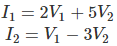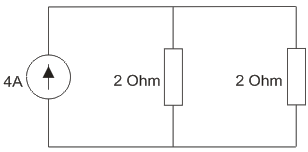# MCQs on Electric Circuits

##### Page 53 of 63. Go to page 1 2 3 4 5 6 7 8 9 10 11 12 13 14 15 16 17 18 19 20 21 22 23 24 25 26 27 28 29 30 31 32 33 34 35 36 37 38 39 40 41 42 43 44 45 46 47 48 49 50 51 52 53 54 55 56 57 58 59 60 61 62 63
01․ A two port network is defined by the relationThe value of Z11 is
2.
-2/11.
-3.
2/11.

Y11 = 2, ΔY = -11; therefore, Z11 = Y11/ΔY.

02․ Which of the following statement is incorrect?
Voltage source is a passive element.
Current source is an active element.
Resistance is a passive element.
Inductance is a passive element.

Voltage source can supply energy to other elements so it is an active element.

03․ Which of the following is not equivalent to watts?
Joules per second.
Amperes/volt.
Ampere2 – Ohm.
Ampere-volts.

Watt is the unit of power and ampere/volt is the unit of conductance.

04․ Three parallel resistive branches are connected across a DC supply. The ratio of the branch resistances are given as R1:R2:R3 :: 2:4:6. The ratio of the branch currents is
6:3:2.
2:3:6.
6:4:2.
2:4:6.

Let I1, I2 and I3 be the branch currents. Voltage drop across each branch is same. So, I1R1 = I2R2 = I3R3. R1:R2:R3 :: 2:4:6. If the common ratio is Z. Then R1 = 2Z, R2 = 4Z and R3 = 6Z. 2ZI1= 4ZI2 = 6ZI3; I1/I2 = 2:1 and I2/I3 = 3:2. So I1:I2:I3 :: 6:3:2

05․ Four resistances 80 Ohm, 50 Ohm, 25 Ohm and R are connected in parallel. Current through 25 Ohm resistance is 4A. Total current of supply is 10A. The value of R will be
66.66.
36.36.
40.25.
76.66.

Voltage drop across each parallel branch = 25×4 = 100V Total current is 10A. So equivalent resistance is 10 Ohm 1/10 = 1/80+1/25+1/50+1/R R = 36.36 Ohm

06․ The Norton equivalent of a network is given as IN = 4A and RN = 2 Ohm. When this circuit is connected across a 2 Ohm load, the current through the load is
1 A.
2 A.
4 A.
0 A.

The connection diagram will be as shown in figure belowSo current through load resistance of 2 Ohm will be 2×4/2 + 2 = 2A.

07․ A particular current is made up of two component : a 10 A and a sine wave of peak value 14.14 A. The average value of current is
zero.
24.14 A.
10 A.
14.14 A.

Average DC current = 10 A. Average AC current = 0 A as it is alternating in nature. Average current = 10 + 0 = 10 A.

08․ Cells are connected in parallel in order to increase
life of the cells.
efficiency.
current capacity.
voltage rating.

The current capacity increases with the increase in number of cells connected in parallel.

09․ The arrangement of 12 cells each of internal resistance 1.5 Ohm to give maximum current in external load resistance of 2 Ohm will be
in 3 parallel rows each row having 4 cells in series.
in 4 parallel rows each row having 3 cells in series.
all 12 in parallel.
all 12 in series.

To give maximum current in external circuit, the internal resistance must be equal to the external resistance. If all the cells are connected in series, the internal resistance will be 18 Ohm. If all the cells are connected in parallel, the internal resistance will be 0.125 Ohm. So the cells must be connected in series – parallel combination. If S is the number of cells connected in series and P be the number of parallel rows, then equivalent resistance is S*1.5/P = 2 So S = 4 and P =3.

10․ A current is said to be alternating when it changes in
magnitude.
direction.
both ‘a’ and ‘b’.
none of these.

An alternating current changes in both magnitude and direction with time.

<<<5152535455>>>